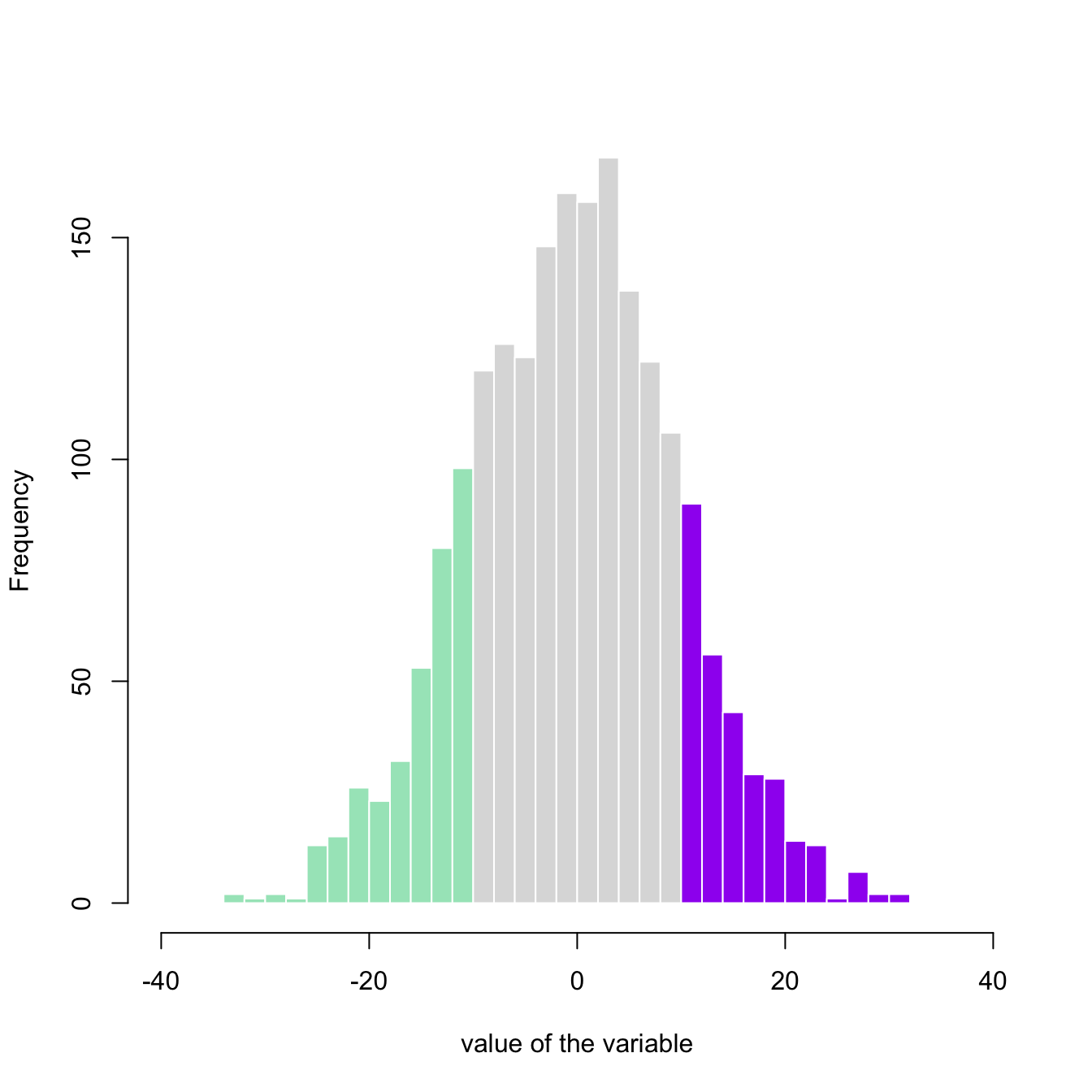# Histogram with colored tail

A histogram displays the distribution of a numeric variable. This posts explains how to color both tails of the distribution in Basic R, without any package. This can be useful to highlight a part of the distribution.

This example demonstrates how to color parts of the histogram. First of all, the `hist` function must be called without plotting the result using the `plot=F` option. It allows to store the position of each bin in an object (`my_hist` here).

Those bin borders are now available in the `\$breaks` slot of the object, what allows to build a color vector using `ifelse` statements. Finally, this color vector can be used in a `plot` call.``````# Create data
my_variable=rnorm(2000, 0 , 10)

# Calculate histogram, but do not draw it
my_hist=hist(my_variable , breaks=40  , plot=F)

# Color vector
my_color= ifelse(my_hist\$breaks < -10, rgb(0.2,0.8,0.5,0.5) , ifelse (my_hist\$breaks >=10, "purple", rgb(0.2,0.2,0.2,0.2) ))

# Final plot
plot(my_hist, col=my_color , border=F , main="" , xlab="value of the variable", xlim=c(-40,40) )``````

Related chart types

## Contact

This document is a work by Yan Holtz. Any feedback is highly encouraged. You can fill an issue on Github, drop me a message on Twitter, or send an email pasting yan.holtz.data with gmail.com.